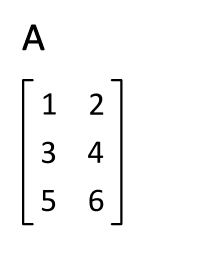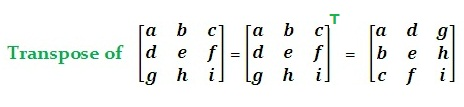## Transpose Matrix Calculator

 Matrix A    3 x 32 x 33 x 23 x 11 x 32 X 22 X 11 X 2 =
 Result: A Transpose =

In linear algebra, the transpose of a matrix A is another matrix AT (also written A′, Atr,tA or At) created by any one of the following equivalent actions:The Transpose Matrix Calculator to convert the matrix A to transpose matrix At

The calculator apply for matrices 3x2,3x3, 3x1,2x3, 1x3, 2x2, 2x1 and 1x2 to transpose the matrix A.

The Fig below shows how to transpose:Thinkcalculator.com provides you helpful and handy calculator resources.